# DAV Class 3 Maths Chapter 7 Worksheet 7 Solutions

The DAV Class 3 Maths Solutions and DAV Class 3 Maths Chapter 7 Worksheet 7 Solutions of Money offer comprehensive answers to textbook questions.

## DAV Class 3 Maths Ch 7 WS 7 Solutions

Question 1.
Find the difference.

(a)Solution:
₹ 50.20

(b)Solution:
₹ 2.52

(c)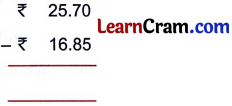Solution:
₹ 8.85(d)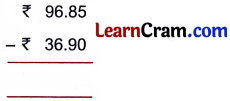Solution:
₹ 59.95

(e)Solution:
₹ 11.78

(f)Solution:
₹ 50.55Question 2.
Arrange in columns and subtract.

(a) ₹ 45.31 from ₹ 80.79
Solution: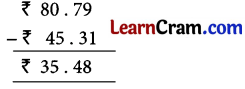(b) ₹ 17.75 from ₹ 34.10
Solution:(c) ₹ 0.07 from ₹ 19.00
Solution: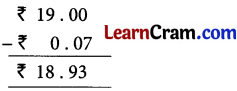(d) 55 paise from ₹ 13.00
Solution: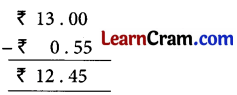### DAV Class 3 Maths Chapter 7 Worksheet 7 Notes

Example:

Subtract ₹ 31.50 from ₹ 93.90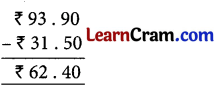The dot remains in the same column in subtraction.